Courses

# Noise Factor And Noise Figure Electronics and Communication Engineering (ECE) Notes | EduRev

## Electronics and Communication Engineering (ECE) : Noise Factor And Noise Figure Electronics and Communication Engineering (ECE) Notes | EduRev

The document Noise Factor And Noise Figure Electronics and Communication Engineering (ECE) Notes | EduRev is a part of the Electronics and Communication Engineering (ECE) Course Communication Theory.
All you need of Electronics and Communication Engineering (ECE) at this link: Electronics and Communication Engineering (ECE)

NOISE FACTOR AND NOISE FIGURE:

An important application of noise temperature is its use in the determination of a component‘s noise factor. The noise factor quantifies the noise power that the component adds to the system when its input noise temperature is T0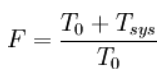The noise factor (a linear term) can be converted to noise figure (in decibels) using: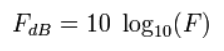If there are multiple noisy components in cascade, the noise temperature of the cascade can be calculated using the Friis equation: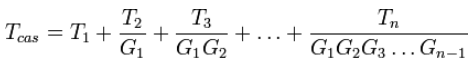where

• T1= noise temperature of the first component in the cascade
• T2= noise temperature of the second component in the cascade
• T3= noise temperature of the third component in the cascade
• G1= noise temperature of the nth component in the cascade
• G2= linear gain of the first component in the cascade
• G3= linear gain of the second component in the cascade
• G4= linear gain of the third component in the cascade
• Gn-1= linear gain of the (n-1) component in the cascade

Components early in the cascade have a much larger influence on the overall noise temperature than those later in the chain. This is because noise introduced by the early stages is, along with the signal, amplified by the later stages. The Friis equation shows why a good quality preamplifier is important in a receive chain

MEASURING NOISE TEMPERATURE:

The direct measurement of a component‘s noise temperature is a difficult process. Suppose that the noise temperature of a low noise amplifier (LNA) is measured by connecting a noise source to the LNA with a piece of transmission line. From the cascade noise temperature it can be seen that the noise temperature of the transmission line (T1) has the potential of being the largest contributor to the output measurement (especially when you consider that LNA‘s can have noise temperatures of only a few Kelvin). To accurately measure the noise temperature of theLNA the noise from the input coaxial cable needs to be accurately known. This is difficult because poor surface finishes and reflections in the transmission line make actual noise temperature values higher than those predicted by theoretical analysis.

Similar problems arise when trying to measure the noise temperature of an antenna. Since the noise temperature is heavily dependent on the orientation of the antenna, the direction that the antenna was pointed during the test needs to be specified. In receiving systems, the system noise temperature will have three main contributors, the antenna (TA ), the transmission line (TL ), and the receiver circuitry ( TR). The antenna noise temperature is considered to be the most difficult to measure because the measurement must be made in the field on an open system. One technique for measuring antenna noise temperature involves using cryogenically cooled loads to calibrate a noise figure meter before measuring the antenna. This provides a direct reference comparison at a noise temperature in the range of very low antenna noise temperatures, so that little extrapolation of the collected data is required.

NOISE FIGURE:

Noise figure (NF) is a measure of degradation of the signal-to-noise ratio (SNR), caused by components in a radio frequency (RF) signal chain. The noise figure is defined as the ratio of the output noise power of a device to the portion thereof attributable to thermal noise in the input termination at standard noise temperature T0 (usually 290 K). The noise figure is thus the ratio of actual output noise to that which would remain if the device itself did not introduce noise. It is a number by which the performance of a radio receiver can be specified.

The noise figure is the difference in decibels (dB) between the noise output of the actual receiver to the noise output of an ―ideal‖ receiver with the same overall gain and bandwidth when the receivers are connected to sources at the standard noise temperature T0 (usually 290 K). The noise power from a simple load is equal to kTB, where k is Boltzmann's constant, T is the absolute temperature of the load (for example a resistor), and B is the measurement bandwidth.

This makes the noise figure a useful figure of merit for terrestrial systems where the antenna effective temperature is usually near the standard 290 K. In this case, one receiver with a noise figure say 2 dB better than another, will have an output signal to noise ratio that is about 2 dB better than the other. However, in the case of satellite communications systems, where the antenna is pointed out into cold space, the antenna effective temperature is often colder than 290 K. In these cases a 2 dB improvement in receiver noise figure will result in more than a 2 dB improvement in the output signal to noise ratio. For this reason, the related figure of effective noise temperature is therefore often used instead of the noise figure for characterizing satellite-communication receivers and low noise amplifiers.

In heterodyne systems, output noise power includes spurious contributions from image-frequency transformation, but the portion attributable to thermal noise in the input termination at standard noise temperature includes only that which appears in the output via the principal frequency transformation of the system and excludes that which appears via the image frequency transformation.

DEFINITION:

The noise factor of a system is defined as: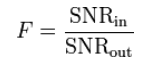where SNRin and SNRout are the input and output power signal-to-noise ratios, respectively. The noise figure is defined as: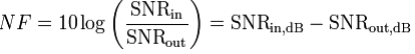where SNRin,dB and SNRout,dB are in decibels (dB). The noise figure is the noise factor, given in dB: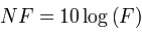These formulae are only valid when the input termination is at standard noise temperature T0, although in practice small differences in temperature do not significantly affect the values.

The noise factor of a device is related to its noise temperature Te :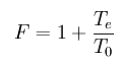Devices with no gain (e.g., attenuators) have a noise factor F equal to their attenuation L (absolute value, not in dB) when their physical temperature equals T0. More generally, for an attenuator at a physical temperature T, the noise temperature is Te = (L − 1)T, giving a noise factor of: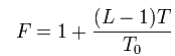If several devices are cascaded, the total noise factor can be found with Friis' Formula: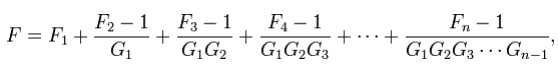where Fn is the noise factor for the n-th device and Gn is the power gain (linear, not in dB) of the n-th device. In a well designed receive chain, only the noise factor of the first amplifier should be significant.

Offer running on EduRev: Apply code STAYHOME200 to get INR 200 off on our premium plan EduRev Infinity!

,

,

,

,

,

,

,

,

,

,

,

,

,

,

,

,

,

,

,

,

,

;